Spatial Dynamics of Human Populations:  Some Basic Models

Ray Holguin
CHASS College Computing
University of California, Riverside

Jason Martinez
California State University
San Bernardino

2005

An Estonian translation has kindly been made available by Paula-Maria Niculescu:

http://www.bildelarstore.se/science/sundimus-suremus-kandevoime-ja-migratsiooni

Fertility, Mortality, Carrying Capacity, and Migration

Developing the model

Virtually all sociological theories regard the number of actors in populations to be a critical cause and effect of other important things.  For example, large numbers of individual persons need to be concentrated before a complex division of labor can emerge; a high level of differentiation can provide productivity gains that allow expansion of population.  Population dynamics are a central part of theories of small groups, communities, informal and formal organizations, whole societies, and the global system.  Models of population dynamics are quite advanced in human demography and ecology.  Many of the insights of these literatures have been applied to other types of populations of social agents (organizations, societies, etc.).

On this page, we are going to develop a very simple model of population dynamics.  We begin with Malthus' (and many others) insight that the numbers in populations tend to increase exponentially in the absence of any environmental limits.  Of course, populations of social agents do not reside in limitless environments.  So, we will next add the idea of "carrying capacity," and model how it limits population growth.  Taken together, these dual forces of growth and limitation make up the classical "logistic" population growth model that is the starting point for almost all demographic/ecological models.

Populations also exist in space, and individuals may migrate from one place to another.  The last part of our basic model development will explore some of the implications of movements of individuals from one population to adjacent populations.  We will examine one very simple theory of migration (there is a large and rich literature of theory and empirical research on this subject).  In our model, there is a normal tendency for some members of one population to migrate to adjacent populations.  When the resources of an area are large (i.e. the carrying capacity is high) relative to the size of the population, however, individuals will be less likely to migrate.  When resources are tight (i.e. population exceeds carrying capacity), the natural tendency for migration is accelerated.  We are going to suppose that the retardation and acceleration of migration based on resource availability are exponential; that is, small deviations of population from carrying capacity have little effect on normal migration, but larger deviations have much greater effects.

A basic demographic model

Figure 1 is a systems dynamics diagram of a very simple population model.  The number of agents in the population at any instant is "N."  "N" can increase or decrease (indicated by the double-headed "flow") and is the accumulation of changes in population that occur over time (dN/dt).

Figure 1.  Population growth in an unlimited environment: Model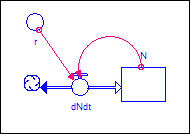The causal theory is really about what causes population to be increasing or decreasing over time; that is, what causes dN/dt.  The actual level of population is simply the accumulation of all past changes.

In our very simple demographic model, we suppose that, in the absence of any limits, there will be a net change in population (r = a surplus of births over deaths, or a surplus of deaths over births) that can be expressed as a probability.  If "r" is a positive number, for example, .05, then there is a 5% chance that each individual in the population will produce one more off-spring in one unit of time than the number of deaths in that interval.  The total net number of off-spring produced, then, is r*N (the probability of any individual reproducing above the death rate,  times the number of individuals).  Depending on the kinds of entities being modeled, different choices of "r" would be made.  We'll suppose that we are examining populations of individual humans, and we'll choose a value of "r" between zero and one.

What will happen to the size of the population when we observe this process acting over time?  Figure 2 shows a simulation of the model dN/dt = r*N, for an initial value of N=10, and r = .05.

Figure 2:  Population growth in an unlimited environment:  Simulation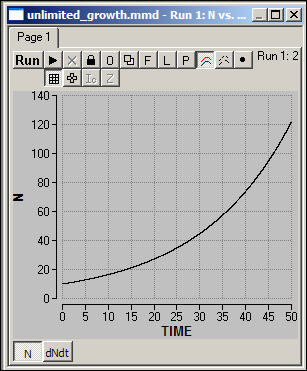The size of the population increases at an increasing rate.  Even though the risk that any one agent has an off-spring in excess of the death rate in any one period of time is a constant, the size of the population "at risk" is steadily increasing.  The total population, therefore, increases exponentially without limit.

Social agents, however, do not live in unlimited resource environments.  At any one time and place, there are limits to how many individuals can be supported by the resources available.  The maximum number of agents that can be supported (at some defined level, usually "subsistence") is the "carrying capacity."  Figure 3 modifies our theory to include the carrying capacity ("k") as an additional cause of over-time change in the rate of change of population.

Figure 3.  Logistic net population growth: Model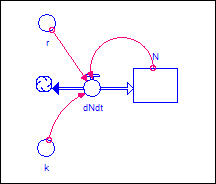One might imagine a number of ways that carrying capacity affects the net reproduction rate.  It may be that when population exceeds the carrying capacity the death rate is increased; it may be that as population grows large relative to the death rate, there is a restriction in fertility.  For our model, we are going to use this equation to describe the relationship:

dN/dt = (r*N)  * (1 - (N/k))

The first part of this is familiar -- it is our exponential growth model.  The second part describes how carrying capacity restricts population growth.  As population approaches carrying capacity (that is as N/k gets closer to one), the right-hand part of the expression becomes closer to zero.  As this happens, less and less of the tendency toward exponential growth is "realized."  Figure 4 shows a simulation of this version of the model.

Figure 4.  Madonna logistic model:  Simulation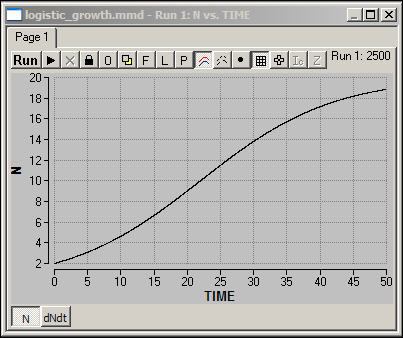When population is low, it tends to increase at an increasing rate.  Beyond a turning point or threshold, however, the growth rate becomes less and less as the carrying capacity is approached.  The result is a smooth "S-shaped" curve called the "logistic" growth curve.

There are many more complex models of basic population dynamics than the simple one we've developed.  Since our main concern is with the effects of spatial coupling of populations in making for complex population dynamics, we're not going to explore these issues here.

Suppose that we had more than one population, and that the populations were adjacent.  Furthermore, suppose that individuals could migrate from one population to the other.  Figure 5 shows a systems dynamics diagram of two populations "coupled" by bi-directional migration.

Figure 5.  Two-region model with migration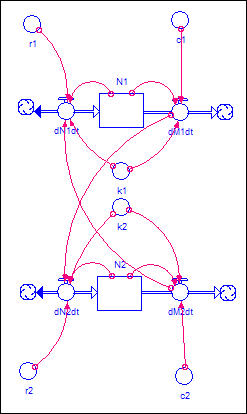There is a basic logistic demographic model operating in each population (N1 and N2).  This time, however, we've shown an additional source of change in each population - dMdt.  This is the rate of migration out of each population.  We also show arrows connecting the out-migration from each population to the growth rate in the other.  This indicates that agents who leave one population are migrating to the other.

Now, we need a theory of migration to determine how many individuals will move from one region to the other.  There are many sophisticated theories, and we will develop only a very simple one here.  We will suppose that some fraction of the population of each area will migrate in each period of time, all else constant.  This fraction is indicated as "c" in Figure 5.  So, the number of persons leaving each population as a result of this "normal" or "frictional" movement is dM/dt = c * N.

When resources are plentiful in a region (i.e. population is less than carrying capacity, N/k < 1) we suppose that "normal" migration is reduced.  When there is scarcity (i.e. population is greater than carrying capacity, N/k > 1), we suppose that "normal" migration is amplified:  dM/dt = (c * N) * (N/k).  We suppose, however, that the effect of wealth or scarcity on the propensity to migrate is not linear.  Small differences of population from carrying capacity do not have much impact on migration, as the motivation to move (or stay) are not strong enough to overcome the "transaction costs" and "uncertainty" involved in moving.  However, as conditions become more extreme (either much greater wealth per capita, or much greater scarcity), we suppose that the response (either the commitment to stay, or the motivation to leave) become stronger at an increasing rate.  We model this by supposing that the effect of N/k on normal migration is in the form of an exponential (in our simple example, we use (N/k)2.

Figure 6 shows one simulation of the two-region model in which the population in region N1 (blue) is initially higher than carrying capacity, and the population in region N2 (red) is initially at carrying capacity.

Figure 6.  Simulation of two-region model with migration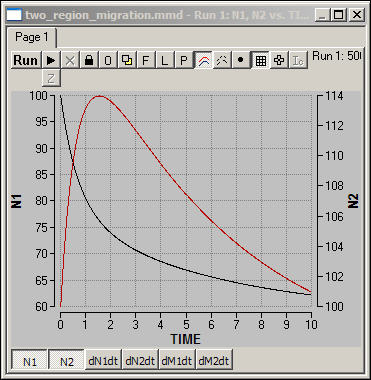Since there is "excess" population in the blue region (N1), there is rapid net migration, initially from N1 to N2.  Since the red region (N2) is already at capacity, it's population is swelled by new immigrants, but then declines as mortality exceeds fertility.  As the model runs, the population of both regions approaches their carrying capacities, and migration rates come closer and closer to "normal."

You might want to download the program and conduct some experiments.  The main factors underlying the dynamics in this model are the sizes of the initial populations, and how far these populations are from carrying capacity.  To get a good grasp on the range of behavior that the theory can predict, you might design a set of experiments:

• What if both regions are above carrying capacity?
• What if both regions are below carrying capacity?
• What if one is above and the other below carrying capacity?
• Do the patterns observed in the three situations above hold true when the populations and carrying capacities of the regions are different from one another and when the regions are the same?

Some simple experiments

Now that we have the basic ideas, we want to expand the model to include some greater spatial complexity.

There are a number of issues that we could explore.  Here are a couple interesting ones that we won't examine; but you're invited to download the model and experiment.

The size of the space, and how boundaries are treated is consequential for dynamics.  For our examples, below, we will look at a very simple 3-by-3 space.  In our model, agents migrate from each space to all available Moore neighbors.  So, "corner" squares have 3 neighbors, edge squares have 5, and central squares have 8.  In our model, the total number of migrants are divided among the available neighbors.  One might, alternatively, chose proportions migrating to each neighbor randomly, or choose a single neighbor and have all migrants move in that direction.

The "coupling topology" (e.g. is migration done in neighborhoods, along a network, or over continuous distances) of the populations is an interesting area to explore.  We'll use the Moore neighborhood approach to space.  A Von Neuman, social network distance, or spatial gradient approach would produce different results.

The two questions we would like to explore, briefly, are what happens when population is not in spatial equilibrium, and what are the effects of non-homogeneous distributions of carrying capacity across space?

Non-homogeneous initial population distribution

Populations often first appear at a single point in a space, and "disperse" by migration throughout the space.  In Figure 7, we've done one experiment with our simple model to try to get a sense of what the spatial dynamics of population dispersion look like.

In the current scenario, we begin with all population concentrated in the central square of a 3-by-3 grid.  All the squares on the grid have equal carrying capacity of 1,000 individuals, and the size of the population in the central square, initially, exceeds this carrying capacity (1,500).  Figure 7 shows the results of a simulation of these initial conditions.

Figure 7.  population movements in 9 square grid, empty except for 1.5 times capacity in center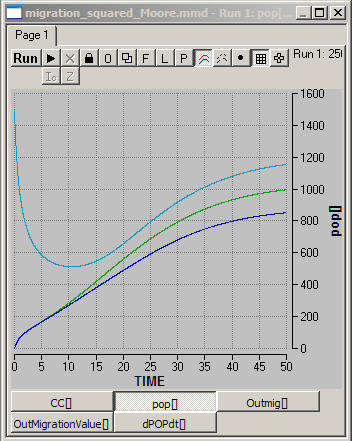The top, light blue, line shows the population trend for the central location.  Since the population there exceeds carrying capacity, there is a lot of out-migration initially.  After a time, out-migration slows as population size declines below carrying capacity; some return migration begins to occur; and internal logistic population growth eventually comes to dominate the dynamics.  Note, however, that the apparent equilibrium population of the central square seems to exceed it's carrying capacity.

The non-central populations are either edges or corners, and we see that the shape of their trends (the green and dark blue lines) are similar, but that the final equilibrium values are not.

• Why does the system reach an equilibrium?
• Why is the equilibrium level of population the same in all spatial areas?  After all, the carrying capacity of all the areas is the same.
• When the system has reached equilibrium, does this mean that there is no longer any migration occurring?
• Why does the first part of the curves look exponential, and the later part of the curves look logistic?  Can you think of different initial conditions that would produce different looking trends?
• Where would you prefer to live?  Where is the level of per capita living highest?
• What would the patterns look like if the grid were much larger?
• What would the patterns look like if the initial population was located in a corner, instead of the center?

A key lesson here is that space matters.  Even though the birth-death-migration model and it's parameters are identical in all spaces, and even though the carrying capacity of the environment is the same in all spatial areas, the "equilibrium" levels of population are not.

Non-homogeneous carrying capacity

Let's consider an alternative scenario where population densities are equal, but carrying capacities are not.  Imagine that we moved 1,000 people to each of the 9 grid squares.  But suppose that only two corner squares had the capacities to support any significant populations.  What will happen?  Figure 8 shows one scenario like this.

Figure 8.  All cells at 1000 population, carrying capacity at 5000 in two corners, 10 elsewhere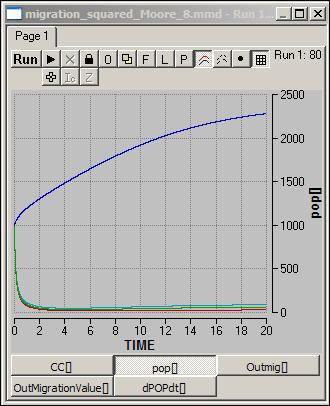What happens, of course, is that people living in squares that have low carrying capacity are going to move out, looking for spaces where the level of living is higher.  Within just a few time steps, the "poor" areas have nearly emptied of population, and population has become concentrated in the two "rich" areas.  From that point on, logistic growth dominates the dynamics in all regions (but operates at very different levels).

You might want to download this program and experiment with creating a larger grid that is a "map" of the levels of resources available across some real geographical space.  What will the equilibrium result for the distribution of population look like?

Directions for development of more sociologically realistic models

The core ideas of the very simple model here are common across a wide range of sociological theories.  The general idea that the spatial distribution and concentration of populations of social agents (people, families, organizations, etc.) depend on net reproduction and migration occurring on a "sub-strate" of carrying capacity is a starting point shared by most theories of social dynamics.

Demographic theory has explored in great detail the relationships of fertility and mortality to carrying capacity (and to other factors, such as distributional inequality).  Human ecology has explored in great detail the relationships between carrying capacity, population, and migration.  More sophisticated treatments of these connections within the spatial framework would be useful directions for future work.

Classical sociological theory also suggests a number of directions for future work that would make the basic model more realistic -- as well as making it a bit more dynamic and complex.

Social organization increases carrying capacity

In the model developed here, "carrying capacity" is treated as a constant.  There are a number of problems with this simplification, and these problems provide opportunities for further work:

The carrying capacity of an area may consist of both renewable and non-renewable resources.  Non-renewable resources must be replaced with substitutable resources (or made irrelevant to survival by technological change), and "carrying capacity" declines as the levels of these resources decline.  Renewable resources are not consumed at constant rates.  Rates of consumption depend on distributional equality, marginal costs of production, and the speed with which resources reproduce.  In many cases, over-use of renewable resources may drive the resources to extinction, resulting in a permanent loss of carrying capacity.

Very importantly, the carrying capacity of spaces for human populations depends very much on the technology available.  The carrying capacity of resource environments can be hugely expanded by the application of technology -- new materials become resources as we learn how to exploit them, and the efficiency of use of resources can be greatly modified by improved technology.  The relationship between population density and level of technological development is an are of considerable theoretical and empirical interest and controversy.  Making "carrying capacity" endogeneous to the model has many interesting theoretical possibilities.

Social organization drives systems beyond sustainable limits

As population increases and concentrates, the division of labor increases, increasing productivity and carrying capacity.  However, this process is ultimately bounded by the physical environment and the level of technological knowledge.  The higher productivity of complex social organization depends on increasingly large investment in infrastructure and coordinative activity.  It may be, however, that there are decreasing marginal  returns in productivity to increased social complexity.

The increasing division of labor and growth of the coordinative component of social structures also re-distributes power in ways that make tendencies toward growth in the coordinative component self-generating.  Many theorists have held that societies may escape collapse for lengthy periods of time by increasing the complexity of organizational coordination; ultimately, however, productivity does not expand at the same rate as overhead, and complex social structures may collapse.  Adding theories of the division of labor and it's consequences to the current model would enable us to add a spatial dimension to macro sociological theories of growth and collapse that is (largely) missing in current work.

Distributional inequalities

A key aspect of the consequences of population growth and increasing division of labor in society is the emergence of class structures that unequally distribute resources.  The response functions of births, deaths, migration, and carrying capacity may be greatly affected by levels of social inequality.

In spatial models like the one here, there is a second form of social inequality that is likely to be very consequential -- inequality between populations.  Differing levels of carrying capacity across space act as powerful "pull" factors in migration.  Our current model treats only the "push" side of the equation.  The simple gravity model developed on another page in this website might be further developed as a way to add "pull" or "attraction" between populations to the model.